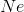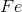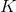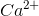# AP Chemistry : Ions

## Example Questions

### Example Question #21 : Elements, Ions, And Isotopes

Which of the following molecules has an O atom with the most negative formal charge?

H2O

(CO3)2–

O2

O3

(CO3)2–

Explanation:

For the carbonate ion, there are 3 resonance structures, and each of the 3 oxygen atoms carries a formal charge of –1 in two out of the three structures. This means that there is a –2/3 charge on each O atom. There are no formal charges in H2O or O2. and in O3, the central O has a positive charge and each outer O has a negative charge, so there is an average charge of –1/2 on the two outer ones.

### Example Question #1 : Ions

What is the formal charge on the N atom in the [NH4]+ ion?

2

-1

0

1

1

Explanation:

N has 5 valence electrons. In the ion, N has 4 bonds, and thus 8 bonding electrons and 0 nonbonding electrons. Thus, formal charge = 5- (1/2)*8 - 0 = 1.

### Example Question #22 : Elements, Ions, And Isotopes

Which of the following ions or atoms is isoelectronic with neon?

Cl-

Na

Mg

O2-

O2-

Explanation:

Neon has an electron configuration of 1s22s22p6, just like O2-. Sodium has one extra electron, while magnesium has two extra electrons, beyond the neon configuration. Finally, the electron configuration for Cl- is 1s22s22p63s23p6. It is one period below flourine; F- would be isoelectric with neon.

### Example Question #22 : Elements, Ions, And Isotopes

Which of the following orders the given elements in terms of increasing electronegativity?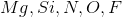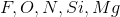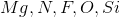Explanation:

Electronegativity increases moving from the left side of the periodic table to the right, and decreases moving down through a group (Excluding the Group 18 noble gases).

Thus the correct answer is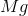(the furthest left), followed by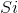,,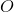, and(the most electronegative element).

### Example Question #1 : Ions

Which of the following ranks the acids from strongest to weakest?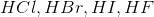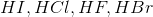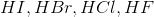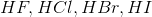Explanation:

Charge stability of halide ions increases with increasing atomic size (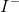is more stable than, which is more stable than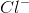, which is more stable than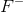).

This is not to be confused with electronegativity trends, which would predict the ordering of acids opposite the correct answer.

Atomic size (and thus the propensity to release protons (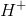) into solution forming anionic halides) increases moving down Group 7, so the correct ordering is:.

This trend is tue, however, keep in mind that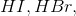and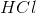are all considered strong acids. This means that they dissociate completely in solution and have negative pKa values.

### Example Question #2 : Ions

Anionic atoms are __________ than their respective neutral atoms because they have __________ electron(s) than their neutral atoms.

smaller . . .  less

larger . . . more

smaller . . .  more

larger . . .  less

larger . . . more

Explanation:

Anions have at least one more electron than their neutral atoms. Adding an electron creates a shielding effect on other electrons in the same shell, decreasing the nuclear charge felt by each valence electron. As a result, the electrons will lie slightly further from the nucleus, corresponding to an increase in atomic radius. If there are no other electrons than the newly added electron in the valence shell, then the added electron was added to the now highest shell, which would significantly increase the size of the atom.

### Example Question #276 : Ap Chemistry

Which of the following is a cation?